# GRE Algebra | Coordinate Geometry | Set 2

• Last Updated : 21 Jan, 2021

Prerequisite – GRE Algebra | Coordinate Geometry

Geometry is the branch of mathematics that is concerned with property and relation between points, lines, solids, surfaces, and high dimensional objects.

Coordinate geometry is the branch of mathematics that deals with two-dimensional figures i.e. x-axis and y-axis in the plane. The X-axis signifies the horizontal plane while Y-axis signifies the vertical plane.

Example : A point is denoted by a combination of both the X-axis and the Y-axis. Points M, N, and L all are points denoted on a two-dimensional plane with X and Y values. M, N, and L have 2, -2, and -3 respectively as their X-axis values while 1.5, -3, and 1.5 respectively as their Y-axis values.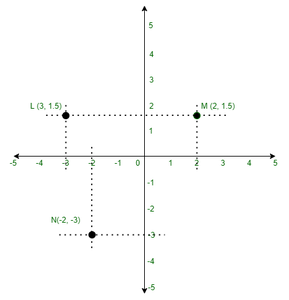Number line :

The number line is a ruler that increases in both directions as per our choice of measurement. On the number line, we can tell where any number is positioned with respect to the origin.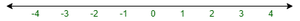Representing a number on a number line. 2 is represented on x-axis 2 points to the right of the origin.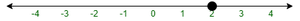The number can be represented on both the axis, X-axis, and Y-axis.

Representing X-axis values :Representing Y-axis values :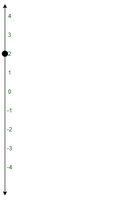Representation of range : If a number is positive we can represent it as shown in the figure below.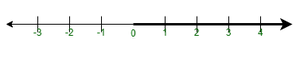Quadrant plane : X and Y values determine the different quadrant the point lies in. Four quadrants are there on basis of different combinations of x and y values.

• 1st Quadrant = X > 0: Y > 0;
• 2nd Quadrant = X < 0: Y > 0;
• 3rd Quadrant = X < 0; Y < 0;
• 4th Quadrant = X > 0; Y < 0;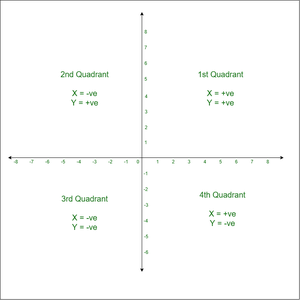Lines in the plane :

The relationship between two x and y has an equation like 2x + 4 = 8y, a line is formed. It can also be generalized in form of :

```y = mx + c
m and c represent constant numbers.
These are also called linear equation```

Slope of the line :

In equation y = mx + c, m is called to the slope of the equation.

`m = Numerator / Denominator = Rise /  Run = Change in Y /Change in X`

Positive Slope : When m > 0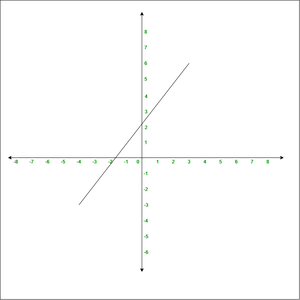Negative Slope : When m < 0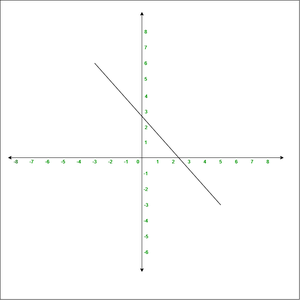Intercept of a line :

A point where a coordinate axis is intersected by a line is called an intercept.

There are two types of intercept :

• X-intercept –
It is where the line intercepts the x-axis. The X intercept is a point on the line where the value of Y is zero. In the diagram shown below (-4, 0) is the -4 is the x-intercept.
• Y-intercept –
It is where the line intercepts the y-axis. The Y-intercept is a point on the line where the value of x is zero. In the diagram shown below (-0, 6) is the 6 is the y-intercept.Distance between two-point:

Pythagoras theorem is used to calculate the distance between two points.
For example, what is the distance between (1,3) and (7,-5)

Steps to find the distance between two points :

• Draw a right triangle connecting the points.
• Find the length of two triangles by calculating the run and rise
• Rise is equal to change in y-axis = 3 – (-5) = 8 (Vertical leg)
• Run is equal to change in x-axis = 7 -1 = 6 (Horizontal leg)• Use Pythagoras theorem to calculate the distance which is the diagonal shown in right angle triangle diagram i.e. c
• 62 + 82 = c2
• 36 + 64 = c2
• 100 = c2
• c = 10

The distance between the two points is 10 units.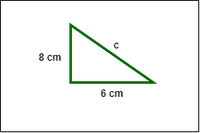Intersection of two lines :

Line in the coordinate plane is the relationship between two x and y has an equation like 2x + 4 = 8y.

```Values of x and y satisfy this equation like (2,1)
2x + 4 = 8y  (2,1)
2*2 + 4 = 8*1
4 + 4 = 8
8 = 8
LHS is equal to RHS
On the other hand point (3,1) doesn't lie on the line.
2*3 + 4 = 8*1
6 + 4 = 8
10 = 8
LHS is not equal to RHS```

So what do we mean by when two lines intersect in the coordinate plane? Two lines intersect in a coordinate plane means that at that particular point, both of the equations that are representing different line holds true for that particular point. That is, the pair of numbers (x,y) that represents the point of intersection solves equations. Finding this point is similar to solving these equations. You can find the intersection by using algebra more easily than by graphing two lines.

```At what point y = 2x - 8 intersect the line represented by 2x + 3y = 10.
Let's solve these equations for x and y.
y = 2x - 8 (equation 1)
2x = 10 - 3y (equation 2)
Putting equation 2 in equation 1
y = 10 -3y - 8
4y = 2
y = 1/2
To find value of x, put value of x in equation 1
1/2 = 2x - 8
1 = 2(2x - 8)
1 = 4x - 16
4x = 1 + 16
4x = 17
x = 17/4
Thus, the point of intersection of two lines is  (17/4, 1/2).```

If lines in a plane do not intersect, then the lines are parallel. In this case, there is NO pair of numbers(x, y) that satisfies both equations at the same time.

Two linear equations can represent a single point, or they can represent parallel lines that never intersect. There is one other possibility: the two-equation might represent the same line. In this case, infinitely many points (x, y) along the line satisfy the two equations( which must actually be the same equation in disguise).

My Personal Notes arrow_drop_up